# Use 4 steps of the Modiﬁed Euler’s method to solve the following diﬀerential equation to t...

Use 4 steps of the Modiﬁed Euler’s method to solve the following diﬀerential equation to t = 2.6, given that y(0) = 1.1. In your working section, you must provide full working for the ﬁrst two steps. To make calculations easier, round the calculations at each step to four decimal places, and provide your ﬁnal answer with four decimal places. dy/ dt = 1.4sin(ty)

## Solutions

##### Expert Solution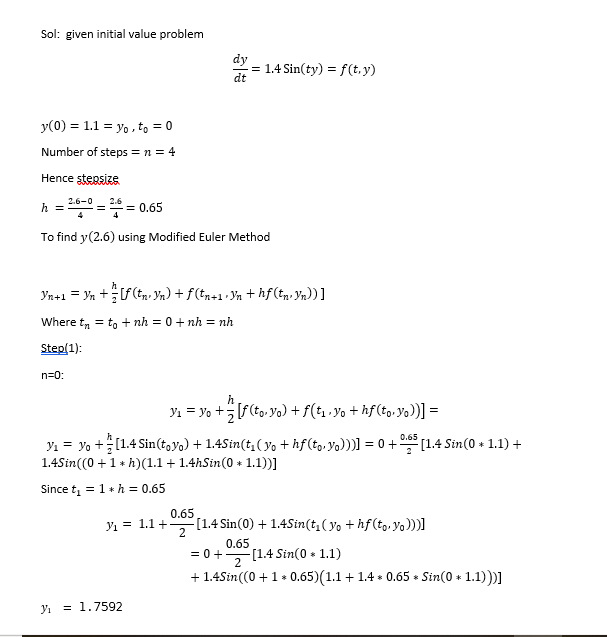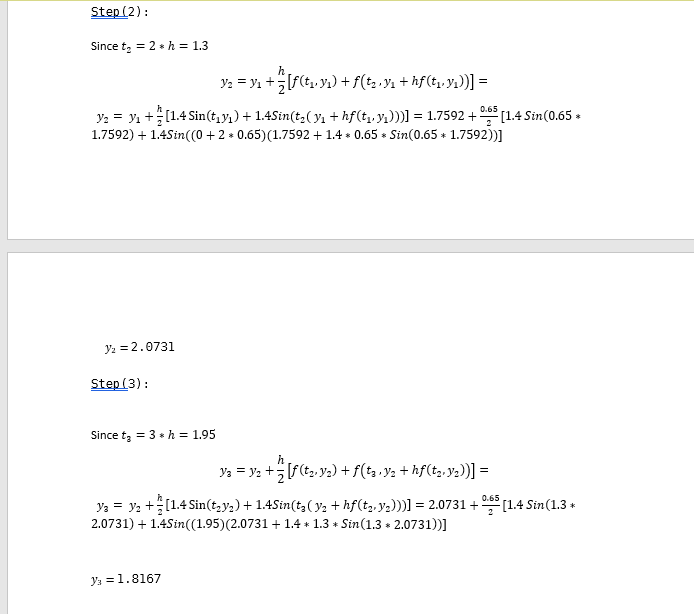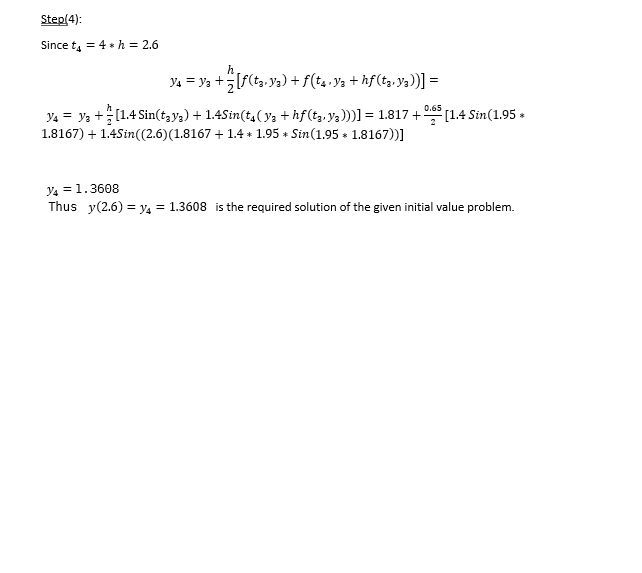##### Expert Solution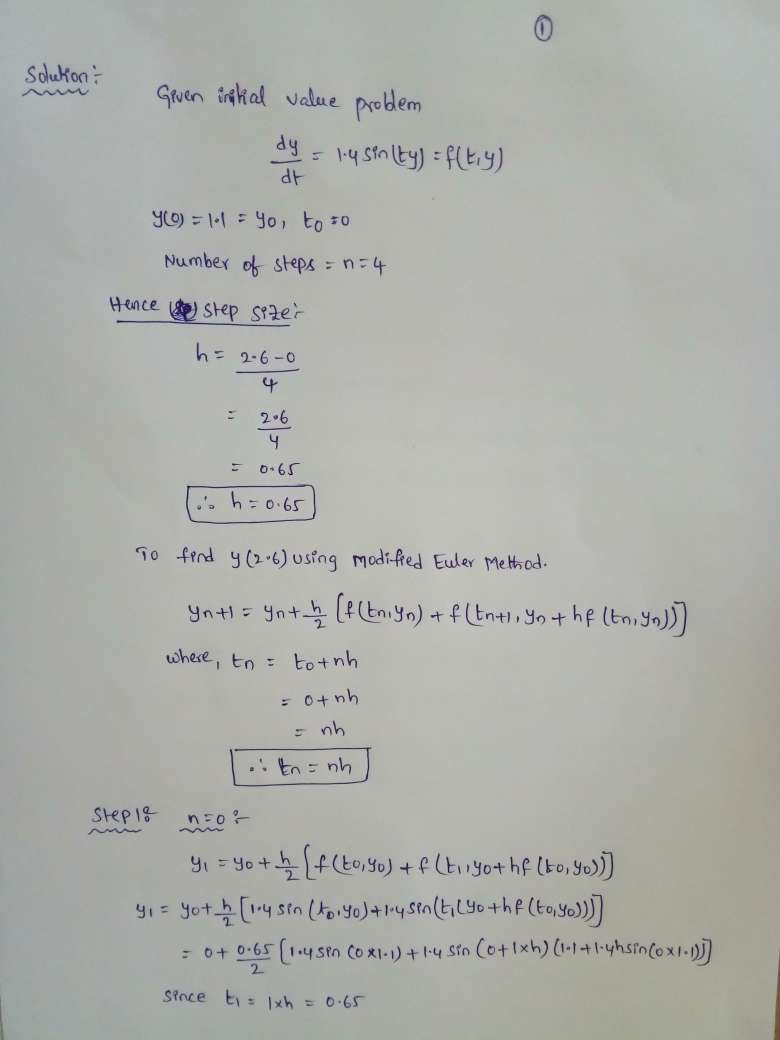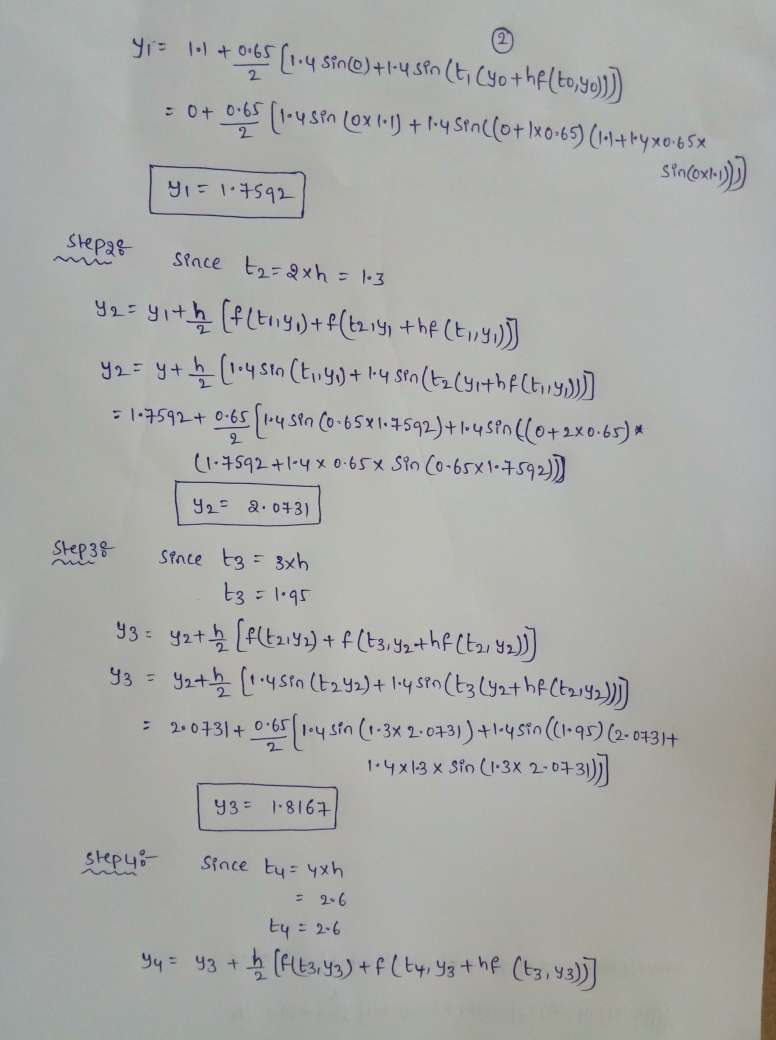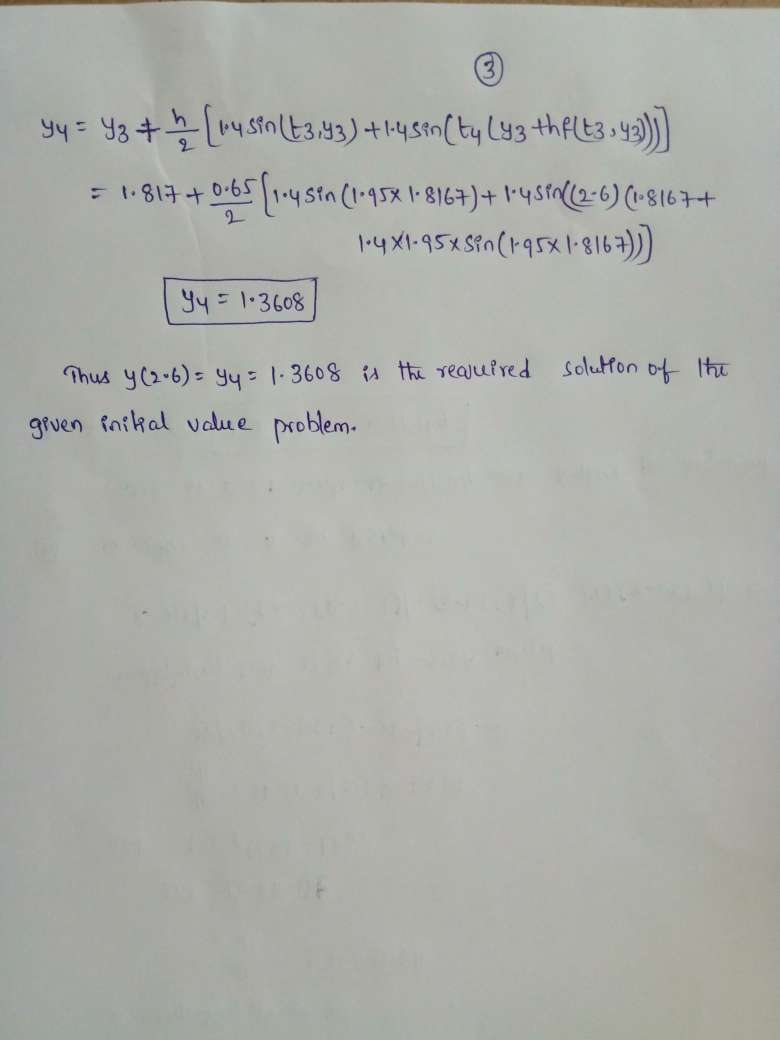## Related Solutions

##### Use 3 steps of the Runge-Kutta (fourth order) method to solve the following diﬀerential equation to...
Use 3 steps of the Runge-Kutta (fourth order) method to solve the following diﬀerential equation to t = 2.4, given that y(0) = 2.3. In your working section, you must provide full working for the ﬁrst step. To make calculations easier, round the tabulated value of y at each step to four decimal places. a) Provide the four K-values that are calculated at the ﬁrst step, with four decimal places. b) Provide your answer for y(2.4) with four decimal places....
##### Use ten steps in Euler’s method to determine an approximate solution for the differential equation y′...
Use ten steps in Euler’s method to determine an approximate solution for the differential equation y′ = x3, y(0) = 0, using a step size Δx = 0.1.
##### Use the Laplace Transform method to solve the following differential equation problem: y 00(t) − y(t)...
Use the Laplace Transform method to solve the following differential equation problem: y 00(t) − y(t) = t + sin(t), y(0) = 0, y0 (0) = 1 Please show partial fraction steps to calculate coeffiecients
##### Euler’s Method Let’s get our hands dirty and actually use Euler’s method to estimate the value...
Euler’s Method Let’s get our hands dirty and actually use Euler’s method to estimate the value of y(2) where y is the solution to the initial value problem y′=y−2x             y(0) = 1 Recall that Euler’s method says: Approximate values for the solution of the initial value problem y′=F(x, y),y(x0) =y0 with step size h, at xn=xn−1+h, are yn=yn−1+hF(xn−1, yn−1) Fill in the table for steps of size h= 0.2. n xn yn=yn-1+0.2F(xn-1,Yn-1 y'=F(xn,yn) 0 0 1 1 .2 2 .4...
##### Use the simplex method to solve the following linear programming problems. Clearly indicate all the steps,...
Use the simplex method to solve the following linear programming problems. Clearly indicate all the steps, the entering and departing rows and columns and rows, the pivot and the row operations used. An investor has up to N\$450,000 to invest in three types of investments. Type A pays 6% annually and has a risk factor of 0. Type B pays 10% annually and has a risk factor of 0.06. Type C pays 12% annually and has a risk factor of...
##### Use the equation given to solve the following problems: 2 KMnO 4 + 16 HCl —>...
Use the equation given to solve the following problems: 2 KMnO 4 + 16 HCl —> 5 Cl 2 + 2 KCl + 2 MnCl 2 + 8 H2O (a) How many moles of HCl are required to react with 35.0 g of KMnO4 ? (b) How many grams of Cl2 will be produced from 3.50 mol of KMnO4 ? (c) To produce 50.0 g of MnCl2 , how many mL of 3.00 M HCl will react? (d) What is...
##### 2. (Improved Euler’s Method ) Second, work out the first three steps by hand. Then approximate...
2. (Improved Euler’s Method ) Second, work out the first three steps by hand. Then approximate y(2) for each of the initial value problems using Improved Euler’s method, first with a step size of h = .1 and then with a step size of h = .05 using the Excel spreadsheet. (a) dy dx = 2xy, y(0) = 1 (b) dy dx = x − y x + 2y , y(0) = 1 (c) dy dx = y + x,...
##### Use Euler method (as explained on the white board) to solve numerically the following ODE: dy/dt=y+t....
Use Euler method (as explained on the white board) to solve numerically the following ODE: dy/dt=y+t. y(0)=1 You can select the step size. Choose n=3. solve this by hand on a paper and with the aid of Matlab.
##### Solve the differential equation. x''(t)+2x'(t)+5x(t) = 2
Solve the differential equation. x''(t)+2x'(t)+5x(t) = 2
##### Solve differential equation by using undetermined coefficient method y^''+2y^'+y= 4 sin2x
Solve differential equation by using undetermined coefficient method y^''+2y^'+y= 4 sin2x